Next: Using an expansion Up: Implementation without gradient Previous: The NonLinear procedure   Contents

The CoeffLinear procedure

This procedure returns a matrix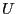of dimension n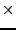m+1 with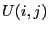equal to the coefficient of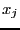in the equation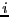while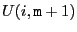is the constant term of the equation. In the previous example this procedure will be:

void CoeffLinear(MATRIX &U)
{
U(1,1)=1; U(1,2)=0.5; U(1,3)=0.3;
U(2,1)=2; U(1,2)=-0.5; U(1,3)=0.2;
}

Jean-Pierre Merlet 2012-12-20